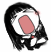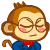﻿ 为人不识C指针，看遍A片也枉然，菜鸟求帮助 | Code Bye

# 为人不识C指针，看遍A片也枉然，菜鸟求帮助

5年前 (2017-04-19) 6105次浏览

aa = (int*)malloc(sizeof(int) * MAXSIZE)就能神奇的把原来的aa指针变量变成一个指针数组，赋值就可以变为aa ，aa，aa等等。

```    int i;
int* y;
y = (int*)malloc(sizeof(int) * 5);
for(i=0;i<5;i++)
{
y[i] = i;
printf("%d\n",y[i]);
}

int i;
int* y;
y = (int*)malloc(sizeof(int) * 5);
for(i=0;i<5;i++)
{
y[i] = &i;
printf("%d\n",*y[i]);
}

int i;
int* y;
y = (int*)malloc(sizeof(int) * 5);
for(i=0;i<5;i++)
{
y[i] = &i;
int* yy = y[i];
printf("%d\n",*yy);
}
```

5

int i;
int* y;
y = (int*)malloc(sizeof(int) * 5);
for(i=0;i<5;i++)
{
y[i] = i;
printf(“%d\n”,y[i]);
}
1、首先，y是一个指向整形数据的指针，假如有一个int型变量 int_data存储着一个整形数据，你可以通过y = &int_data来使y指向变量int_data的地址，此时y是一个地址，你要想查看这个地址上的值，就得通过*y的形式。
2、y = (int*)malloc(sizeof(int) * 5);这句话的可以理解为你申请了一块内存，该内存的大小正好可以保存5个整形数据，并且y指向了该内存的首地址。这5个整形数据对应的内存地址分别是y, y+1, y+2, y+3, y+4，他们也就是对应着&y, &y, &y, &y, &y。

20

y = (int*)malloc(sizeof(int) * 5);

y[i] = *(y+i)

5

《深入理解C指针》

5理解讨论之前请先学会怎么样观察多用小脑和手，少用大脑、眼睛和嘴，会更快地学会编程！

VC调试时按Alt+8、Alt+7、Alt+6和Alt+5,打开汇编窗口、堆栈窗口、内存窗口和寄存器窗口看每句C对应的汇编、单步执行并观察相应堆栈、内存和寄存器变化，这样过一遍不就啥都明白了吗。

（Turbo C或Borland C用Turbo Debugger调试,Linux或Unix下用GDB调试时,看每句C对应的汇编并单步执行观察相应内存和寄存器变化。）

“学习用汇编语言写程序”

“VC调试(TC或BC用TD调试)时按Alt+8、Alt+7、Alt+6和Alt+5,打开汇编窗口、堆栈窗口、内存窗口和寄存器窗口看每句C对应的汇编、单步执行并观察相应堆栈、内存和寄存器变化，这样过一遍不就啥都明白了吗。
（Linux或Unix下可以在用GDB调试时,看每句C对应的汇编并单步执行观察相应内存和寄存器变化。）

30

Quote: 引用:

y = (int*)malloc(sizeof(int) * 5);

y[i] = *(y+i)

```int SeqList_Insert(SeqList* list, SeqListNode* node, int pos) // O(n)
{
TSeqList* sList = (TSeqList*)list;
int ret = (sList != NULL);
int i = 0;

ret = ret && (sList->length + 1 <= sList->capacity);
ret = ret && (0 <= pos);

if( ret )
{
if( pos >= sList->length )
{
pos = sList->length;
}

for(i=sList->length; i>pos; i--)
{
sList->node[i] = sList->node[i-1];
}

sList->node[i] = (TSeqListNode)node;
关键的这句用的指针赋值，在main函数里面的调用是SeqList_Insert(list, &i, 0);
sList->length++;
}

return ret;
}
```

y[i] = &i; 得到的i的地址转换成的int值，在C++里不允许这样的隐式转换

CodeBye 版权所有丨如未注明 , 均为原创丨本网站采用BY-NC-SA协议进行授权 , 转载请注明为人不识C指针，看遍A片也枉然，菜鸟求帮助

[1034331897@qq.com]